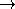### Definition

The concept MinQuadrilateralTraits_2 defines types and operations needed to compute minimum enclosing quadrilaterals of a planar point set using the functions min_rectangle_2, min_parallelogram_2 and min_strip_2.

### Types

 MinQuadrilateralTraits_2::Point_2 type for representing points. MinQuadrilateralTraits_2::Vector_2 type for representing vectors. MinQuadrilateralTraits_2::Direction_2 type for representing directions. MinQuadrilateralTraits_2::Line_2 type for representing lines. MinQuadrilateralTraits_2::Rectangle_2 type for representing (not necessarily axis-parallel) rectangles. MinQuadrilateralTraits_2::Parallelogram_2 type for representing parallelograms. MinQuadrilateralTraits_2::Strip_2 type for representing strips, that is the closed region bounded by two parallel lines.

### Predicates

 MinQuadrilateralTraits_2::Equal_2 a model for Kernel::Equal_2. MinQuadrilateralTraits_2::Less_xy_2 a model for Kernel::Less_xy_2. MinQuadrilateralTraits_2::Less_yx_2 a model for Kernel::Less_yx_2. MinQuadrilateralTraits_2::Has_on_negative_side_2 a model for Kernel::Has_on_negative_side_2. MinQuadrilateralTraits_2::Compare_angle_with_x_axis_2 a model for Kernel::Compare_angle_with_x_axis_2. MinQuadrilateralTraits_2::Area_less_rectangle_2 AdaptableFunctor op: Rectangle_2  × Rectangle_2bool. op(r1,r2) returns true, iff the area of r1 is strictly less than the area of r2. MinQuadrilateralTraits_2::Area_less_parallelogram_2 AdaptableFunctor op: Parallelogram_2  × Parallelogram_2bool. op(p1,p2) returns true, iff the area of p1 is strictly less than the area of p2. MinQuadrilateralTraits_2::Width_less_strip_2 AdaptableFunctor op: Strip_2  × Strip_2bool. op(s1,s2) returns true, iff the width of s1 is strictly less than the width of s2.

The following type is used for expensive precondition checking only.

### Constructions

 MinQuadrilateralTraits_2::Construct_vector_2 a model for Kernel::Construct_vector_2. MinQuadrilateralTraits_2::Construct_vector_from_direction_2 AdaptableFunctor op: Direction_2Vector_2. op(d) returns a vector in direction d. MinQuadrilateralTraits_2::Construct_perpendicular_vector_2 a model for Kernel::Construct_perpendicular_vector_2. MinQuadrilateralTraits_2::Construct_direction_2 a model for Kernel::Construct_direction_2. MinQuadrilateralTraits_2::Construct_opposite_direction_2 a model for Kernel::Construct_opposite_direction_2. MinQuadrilateralTraits_2::Construct_line_2 a model for Kernel::Construct_line_2. MinQuadrilateralTraits_2::Construct_rectangle_2 Function class op: Point_2  × Direction_2  × Point_2  × Point_2  × Point_2Rectangle_2. If the points p1,p2,p3,p4 form the boundary of a convex polygon (oriented counterclockwise), op(p1,d,p2,p3,p4) returns the rectangle with one of the points on each side and one sides parallel to d. MinQuadrilateralTraits_2::Construct_parallelogram_2 Function class op: Point_2  × Direction_2  × Point_2  × Direction_2  × Point_2  × Point_2Rectangle_2. If the points p1,p2,p3,p4 form the boundary of a convex polygon (oriented counterclockwise), op(p1,d1,p2,d2,p3,p4) returns the parallelogram with one of the points on each side and one side parallel to each of d1 and d2. MinQuadrilateralTraits_2::Construct_strip_2 Function class op: Point_2  × Direction_2  × Point_2Strip_2. op(p1,d,p2) returns the strip bounded by the lines through p1 resp. p2 with direction d.

### Operations

template < class OutputIterator >
OutputIterator
 t.copy_rectangle_vertices_2 ( const Rectangle_2& r, OutputIterator o)
const
copies the four vertices of r in counterclockwise order to o.

template < class OutputIterator >
OutputIterator
 t.copy_parallelogram_vertices_2 ( const Parallelogram_2& p, OutputIterator o)
const
copies the four vertices of p in counterclockwise order to o.

template < class OutputIterator >
OutputIterator
 t.copy_strip_lines_2 ( const Strip_2& s, OutputIterator o)
const
copies the two lines bounding s to o.

Additionally, for each of the predicate and construction functor types listed above, there must exist a member function that requires no arguments and returns an instance of that functor type. The name of the member function is the uncapitalized name of the type returned with the suffix _object appended. For example, for the functor type Construct_vector_2 the following member function must exist:

 Construct_vector_2 t.construct_vector_2_object () const